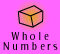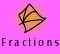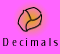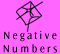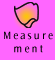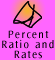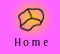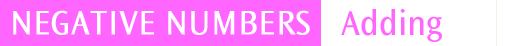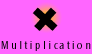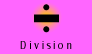| Using the annihilation model | Movies of addition of directed numbers |
| Quick quiz |

Using the annihilation model

We will use the annihilation model to give meaning to the addition of directed numbers. To refresh how we use the model, let's look at how some integers are represented. This model is introduced in Meaning and Models. Recall that any number can be represented in many ways.

 Collection Model Symbol Thinking +1, +1, +1, +1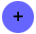+4 Four positive chips represent +4 -1, -1, -1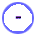-3 Three negative chips represent -3. -1, +1, -1, -1, +1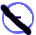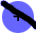-1 Two +1s and three -1s represent -1, since one negative chip and one positive chip annihilate one another, leaving one negative.

The operation of addition is modelled by combining the collections of chips.

Example 1: 5 + 3

5 + 3 can be represented as 5 positive chips plus 3 positive chips, which when combined, gives 8 positive chips.+=+5 + (+3) = +8

Example 2: (-5) + 3

(-5) + 3 is represented as a collection of 5 negative chips combined with a collection of 3 positive chips. When we put the collection together we can see that 3 positive chips and 3 negative chips will annihilate each other, leaving 2 negative chips.+=-5 + (+3) = -2

Example 3: 5 + (-3)

When we add -3 to 5 we can see that 3 positive chips and 3 negative chips annihilate each other, and we are left with 2 positive chips.+=+5 + (-3) = +2

This example shows how the addition of a negative number is the same as subtraction of a positive number. We have added 3 negative chips which is the same as taking away 3 positive chips. We can show this using the model below:

Example 4: 5 - +3=+5 - (+3)
= +2

 To add a negative number, you can subtract the positive value of the number Example: 5 + (-3) = 5 - (+3) = 2 If a and b are any integers, then a + (-b) = a - (+b)

Example 5: (-5) + (-3)+=-5 + (-3) = -8

We can draw a table of basic addition facts to show the patterns that form when we add positive and negative integers.

 + -5 -4 -3 -2 -1 0 +1 +2 +3 +4 +5 +5 0 1 2 3 4 5 6 7 8 9 10 +4 -1 0 1 2 3 4 5 6 7 8 9 +3 -2 -1 0 1 2 3 4 5 6 7 8 +2 -3 -2 -1 0 1 2 3 4 5 6 7 +1 -4 -3 -2 -1 0 1 2 3 4 5 6 0 -5 -4 -3 -2 -1 0 1 2 3 4 5 -1 -6 -5 -4 -3 -2 -1 0 1 2 3 4 -2 -7 -6 -5 -4 -3 -2 -1 0 1 2 3 -3 -8 -7 -6 -5 -4 -3 -2 -1 0 1 2 -4 -9 -8 -7 -6 -5 -4 -3 -2 -1 0 1 -5 -10 -9 -8 -7 -6 -5 -4 -3 -2 -1 0

 A negative number added to a negative number results in a negative number. (-a) + (-b) = -(a + b)

Write down some more patterns that you can see.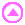Movies of addition of directed numbers

When we are adding and subtracting many directed numbers we sometimes put brackets around the negative numbers so that the example is easier to read.

For Example,

-6 + -7 - -9 + -21 - -4 - 3 + -12 is better written as,

-6 + (-7) - (-9) + (-21) - (-4) - 3 + (-12)

Grouping the number with its negative sign makes it clearer to see what is going on and leaves less chance to make mistakes.

Example 6: movie - using the annihilation model, (-10) + 6

Example 7: movie - using the annihilation model, -14 + 11 + (-6) + (-7) + 5

Example 8: movie - using the number line model, 2 + 3 + (-3) = 2

Quick quiz

 1. Using the annihilation model, or another model if you prefer, find answers to the following examples: a) 6 + (-3) b) -7 + 4 c) -8 + (-5) d) -12 + (-3) 2. Without using a model find answers to the following examples: a) -14 + 6 b) -8 + 10 c) -22 + (-13) d) -7 + (-14) e) -15 + 5 + (-8) + (-7) + 10 f) 13 + -9 + (-11) - 3 g)-22 + (-19) + 4 + (-6) + 2 h) -10 + (-11) + 1 + 2 - 3 3. Heavy rain in the catchment area of the local water reservoir has filled it during the winter. Since then, after a very hot summer, the water level dropped by 5 metres. Subsequent autumn rain has now raised the water level by 1 metre. What is the current level of the reservoir?

'Talking through' questions

The 'talking through' questions and answers below have been provided to enable you to see how an 'expert' might tackle these questions. The annihilation model has been used in the explanations where appropriate.

 1. Find the answer to - 4 + 9 This reads, negative four plus positive nine. Using the annihilation method, we have 4 negative chips and 9 positive chips. When 4 negative chips annihilate 4 of the positive chips we are left with 5 positive chips. So, - 4 + 9 = +5 or just 5 2. Find the answer to - 6 + (- 5) This reads, negative six plus negative five. Using the annihilation method, we have 6 negative chips and another 5 negative chips. Because we have no positive chips we cannot annihilate any chips. We just have a total of 11 negative chips. So, - 6 + (- 5) = - 11